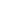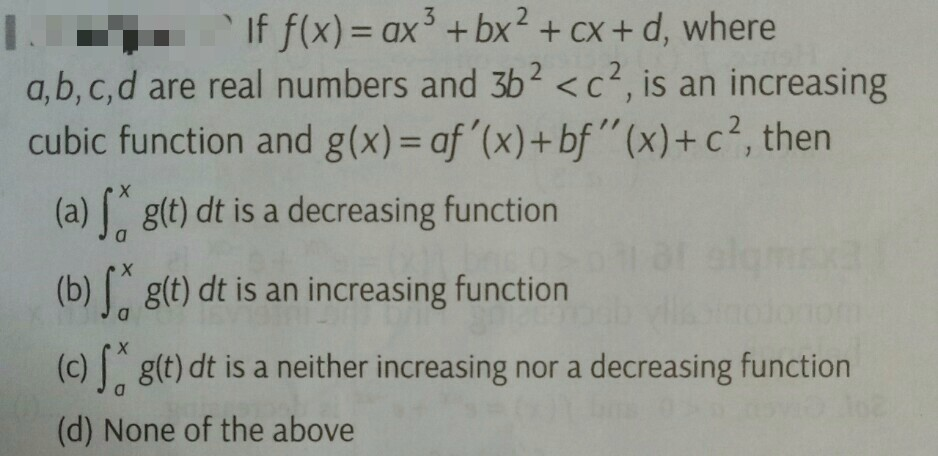Flat 50% off

Ends in

•• 12th
•• Mathematics
• Back to Doubt Clearing

## Pls solve it If $f(x)=ax^3+bx^2+cx+d,$where a,b,c,d are real numbers and $3b^2<c^2,$ is an increasing cubic function and g(x) $=af'(x)+bf''(x) +c^2,then$ (a) $\int^x_a$ g(t) dt is a decreasing function (b)  $\int^x_a$g(t) dt is an increasing function (c) $\int^x_a$ g(t) dt is a neither increasing nor a decreasing function (d) None of the above